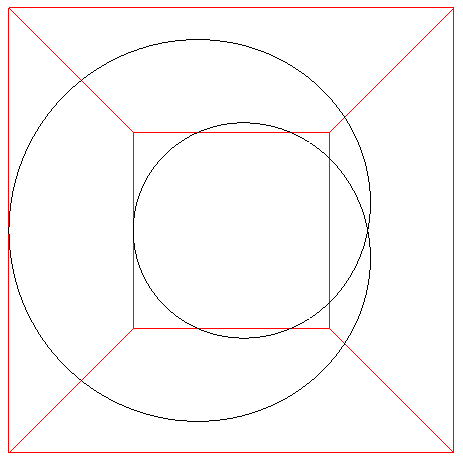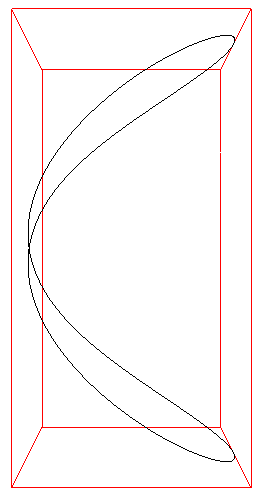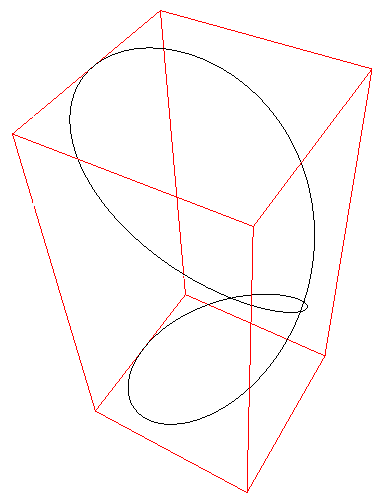# Viviani Curve

By Paul Bourke
January 2001
x = r (1 + cos(t))
y = r sin(t)
z = 2 r sin(t/2)
-2 pi < t < 2 piIntersection curve of the cylinder (x - r)2 + y2 = r2 and the sphere x2 + y2 + z2 = (2 r)2.